Skip to content
{{ message }}

# python-visualization / folium

Have a question about this project? Sign up for a free GitHub account to open an issue and contact its maintainers and the community.

By clicking “Sign up for GitHub”, you agree to our terms of service and privacy statement. We’ll occasionally send you account related emails.

Already on GitHub? Sign in to your account

# Add contour map functionality #958

Open
opened this issue Sep 10, 2018 · 12 comments
Open

# Add contour map functionality#958

opened this issue Sep 10, 2018 · 12 comments
Labels

## Comments

###tjansson60 commented Sep 10, 2018

 Dear all I would like to make a map of weather-like data as temperature or pressure on a folium map similar to the plot below (from https://cdoovision.com/us-temperature-contour-map/):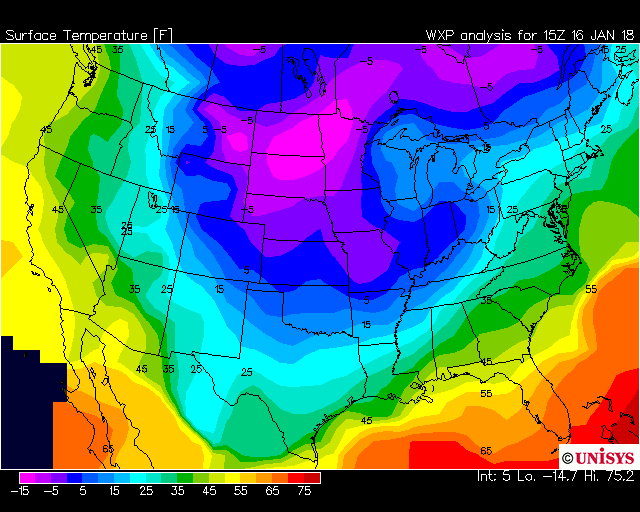As it can be seen it is not a choropleth map as the contours go through the states and it is not a heatmap as I do not care how many measurements underlies this. The data I have is not gridded, but I have many individual measurements semi-randomly scattered over a large area. Is this possible in folium currently in any way? The text was updated successfully, but these errors were encountered:

###jtbaker commented Sep 10, 2018 • edited

 This is a pretty broad question. I think it needs to be reframed in terms of, what does your data look like? There are two methods of data classification for pretty much all GIS - Vector and Raster data. Vector A collection of one or more vertices to form some sort of geometric shape "feature" we can display on a two dimensional surface like a map on our screens - these usually take the form of points, lines, polygons, or multipolygons. This format is suited to data that is either continous or discrete in nature. Raster A grid or matrix array of values on a coordinate plane. You can think of these as "pixels" on a screen, with each pixel having a quantitative "value". They can vary in terms of their resolution, and the area that they cover. This format is better suited to data which is continuous in nature. They're fundamentally different types of data, each with their own strengths and weaknesses. here's some more reading on this subject. The map you posted could easily be plotted as a choropleth map if each of the bands was classified as a polygon with some sort of data classification based on which to color it. But I think in general natural science/physical geography mapping tends to use rasters more commonly. `folium` is capable of plotting both types of data - once you figure out what sorts of data that you have access to, you probably want to start with either the `folium.features.GeoJson` class for vector, or `folium.raster_layers.ImageOverlay` class for raster. There are examples of each in the example notebook section. Hope this helps!

###tjansson60 commented Sep 11, 2018

 Hi Jason Thank for the detailed answer for my perhaps imprecise question. I have collection of semi-randomly distributed vector points for a selection of vehicles where I have (speed, latitude, longitude). I wish to make a contour map of speeds over a folium interactive map. I am not interested in any sectioning of the geografic map in municipalities, zip number or parishes, so a choropleth map is not the solution. Individual points can be also be noisy and they are not interesting by themselves. So what I was looking for was a simple way to superimpose a contour plot on a folium map. In this example a contour map is superimposed on a basemap and the underlying scattered individual points can also be seen: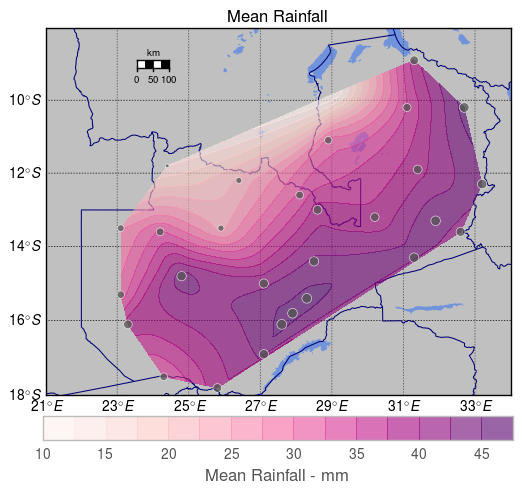(from: https://stackoverflow.com/questions/26872337/how-can-i-get-my-contour-plot-superimposed-on-a-basemap) So I think I understand I could make the gridded contour my self and plot on top of the folium map as a raster layer, but what I was really hopping for what that there was a builtin method that just took the data and some parameters and did it automatically. :) Kind regards Thomas Jansson

###Conengmo commented Sep 11, 2018

 Hi Thomas, Interesting question, but as far as I know this is currently not included in folium. Would be cool to have it though! We use Leaflet under the hood, so maybe you can check if there is any Leaflet plugin that does what you want. We can than implement that plugin in folium.

###tjansson60 commented Sep 11, 2018

 Hi Frank That's the thing. I have grown so accustomed to folium more less being able to do everything I want with very few lines of code, so I think it would make a great addition. :) I didn't find anything in the official leaflet docs, but I did find this interesting article on creating just this kind of plot in R using their leaflet wrapper. So I am guessing their code could be wrapped in a helper function in folium:

###ocefpaf commented Sep 11, 2018 • edited

 @tjansson60 here is what `folium` can do so far: http://nbviewer.jupyter.org/gist/ocefpaf/78095b6e5d22723aae3bf05e5600e165 You'll note that we are lacking: An easy way to add the legend for the color values. We can generate a raster image in the raster example and add as a float image but that is a "hack." A nice way to do the vector option directly from the GeoJSON created by `mplleaflet`, at the moment the color info in there but it is ignored.

###tjansson60 commented Sep 11, 2018

 @ocefpaf Thanks for the example.

###Conengmo commented Sep 17, 2018

 Cool example @ocefpaf. Would be interesting to find a way to implement this in folium in a sensible way. I couldn't find any Leaflet code that does contour maps, so we're on our own.

###ocefpaf commented Sep 17, 2018

 I couldn't find any Leaflet code that does contour maps, so we're on our own. Yep 😄 Would be interesting to find a way to implement this in folium in a sensible way The idea if using `mplleaflet` to plot matplotlib GeoJson is one that I wanted to do for a while. That would cover the vector version of the problem Regarding raster, I guess that is relatively easy to do and I'm not inclined to add a built-in support for it beyond what we already have. The issue with the legend remains though. We need to investigate how to do that properly.

###tjansson60 commented Oct 4, 2018

 A little update from my side: I now have something working and I have written an example below to illustrate this. I hope others perhaps can use this until a real feature is available in folium. As I have written before I have grown accustomed to everything being very simple in folium, so I think a built-in contour map function would be a great addition. :)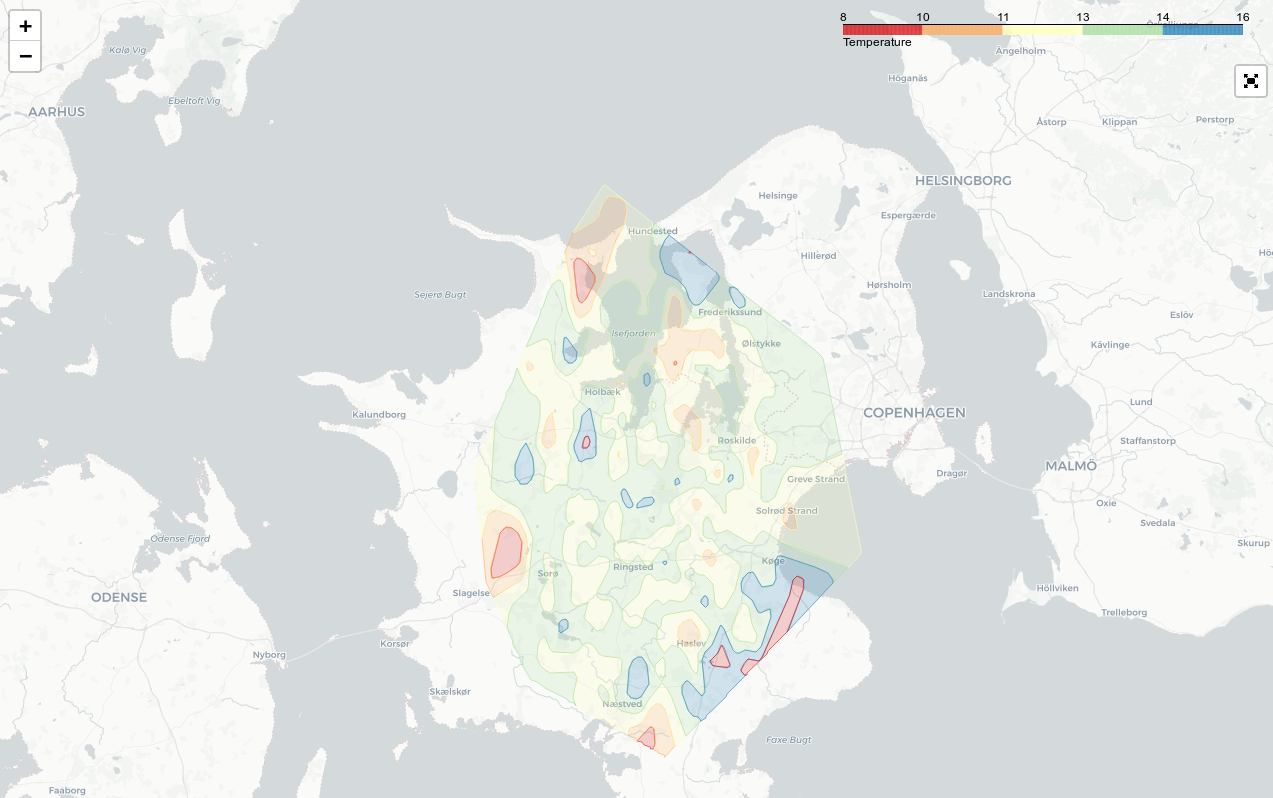```#!/usr/bin/env python # -*- coding: utf-8 -*- import numpy as np import pandas as pd import folium import branca from folium import plugins import matplotlib.pyplot as plt from scipy.interpolate import griddata import geojsoncontour import scipy as sp import scipy.ndimage # Setup temp_mean = 12 temp_std = 2 debug = False # Setup colormap colors = ['#d7191c', '#fdae61', '#ffffbf', '#abdda4', '#2b83ba'] vmin = temp_mean - 2 * temp_std vmax = temp_mean + 2 * temp_std levels = len(colors) cm = branca.colormap.LinearColormap(colors, vmin=vmin, vmax=vmax).to_step(levels) # Create a dataframe with fake data df = pd.DataFrame({ 'longitude': np.random.normal(11.84, 0.15, 1000), 'latitude': np.random.normal(55.55, 0.15, 1000), 'temperature': np.random.normal(temp_mean, temp_std, 1000)}) # The original data x_orig = np.asarray(df.longitude.tolist()) y_orig = np.asarray(df.latitude.tolist()) z_orig = np.asarray(df.temperature.tolist()) # Make a grid x_arr = np.linspace(np.min(x_orig), np.max(x_orig), 500) y_arr = np.linspace(np.min(y_orig), np.max(y_orig), 500) x_mesh, y_mesh = np.meshgrid(x_arr, y_arr) # Grid the values z_mesh = griddata((x_orig, y_orig), z_orig, (x_mesh, y_mesh), method='linear') # Gaussian filter the grid to make it smoother sigma = [5, 5] z_mesh = sp.ndimage.filters.gaussian_filter(z_mesh, sigma, mode='constant') # Create the contour contourf = plt.contourf(x_mesh, y_mesh, z_mesh, levels, alpha=0.5, colors=colors, linestyles='None', vmin=vmin, vmax=vmax) # Convert matplotlib contourf to geojson geojson = geojsoncontour.contourf_to_geojson( contourf=contourf, min_angle_deg=3.0, ndigits=5, stroke_width=1, fill_opacity=0.5) # Set up the folium plot geomap = folium.Map([df.latitude.mean(), df.longitude.mean()], zoom_start=10, tiles="cartodbpositron") # Plot the contour plot on folium folium.GeoJson( geojson, style_function=lambda x: { 'color': x['properties']['stroke'], 'weight': x['properties']['stroke-width'], 'fillColor': x['properties']['fill'], 'opacity': 0.6, }).add_to(geomap) # Add the colormap to the folium map cm.caption = 'Temperature' geomap.add_child(cm) # Fullscreen mode plugins.Fullscreen(position='topright', force_separate_button=True).add_to(geomap) # Plot the data geomap.save(f'data/folium_contour_temperature_map.html')```

###meteoDaniel commented Oct 10, 2018

 I suggest to transform your 2d grib data to tiles. Than you could load the data as a tile layer to your map. This is much faster than plotting geojson data or similar to the map.

###AndreasLuckert commented May 14, 2020

 Hey @meteoDaniel , how would you go about doing what you mentioned in your last comment? Could you please give a minimal example of how to transform 2D-grid data to tiles, maybe simply based on @tjansson60 's example above? At least, I admit I don't know what you mean and therefore I'd have to stick with the `geojson-data method`.

###headfox23 commented Apr 3, 2021

 hello all, i used the approach of @tjansson60 which works very well.. But with very irregular data the contours are not very presice and cause also strange "blobs":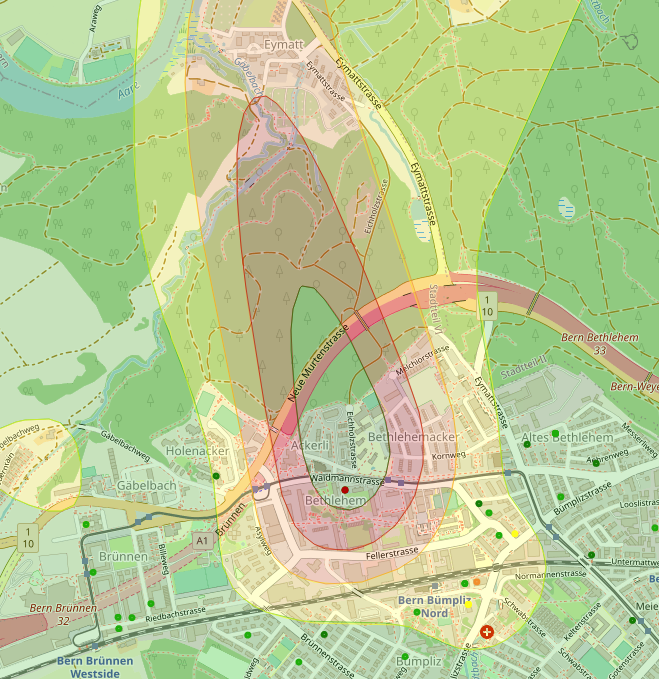And a lot of points are "out" of the right level...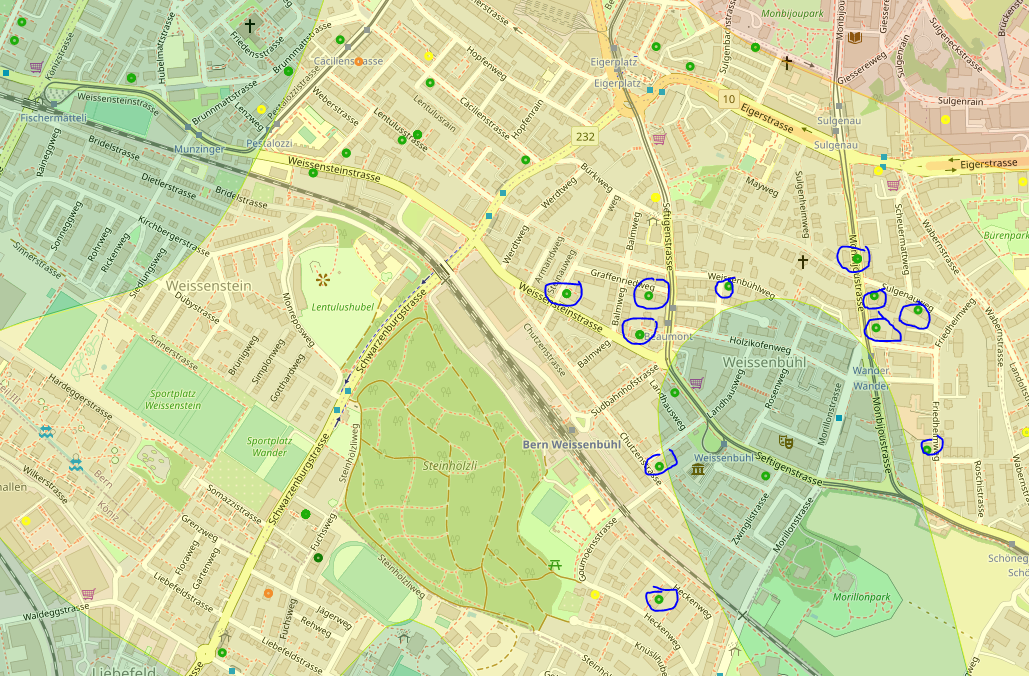Thats the code i used: ```def plotHeatMap(df): print("=== Plotting data") # Prepare Map # Remove nulls df = df[df.latitude.notnull()] df = df[df.longitude.notnull()] df = df[df.roomGrossPrice.notnull()] # remove outliers q_hi = df["roomGrossPrice"].quantile(0.99) df = df[(df["roomGrossPrice"] < q_hi)] x_start = (df['latitude'].max() + df['latitude'].min()) / 2 y_start = (df['longitude'].max() + df['longitude'].min()) / 2 start_coord = (x_start, y_start) # Setup colormap colors = ['#147d05', '#1ead0a', '#ffff17', '#f28e2c', '#b30003'] vmin = df["roomGrossPrice"].min() vmax = df["roomGrossPrice"].max() levels = len(colors) cm = branca.colormap.LinearColormap(colors, vmin=vmin, vmax=vmax).to_step(levels) map = folium.Map(location=start_coord, zoom_start=14) addContourf(map, df, colors, vmin, vmax) for index, item in df.iterrows(): loc = tuple([item["latitude"], item["longitude"]]) folium.Circle( location=loc, radius=5, fill=True, popup="RoomPrice:
" + str(item["roomGrossPrice"]) + "
Rooms:
" + str(item["rooms"]) + "
ID:
" + str(item["id"]), color=cm(item["roomGrossPrice"]), # fill_opacity=0.7 ).add_to(map) cm.caption = 'Room Price' map.add_child(cm) folium.LayerControl().add_to(map) map.save('test.html') print("=== Plotting data finished") def addContourf(map, df, colors, vmin, vmax): print("=== adding Contourf") # The original data x_orig = np.asarray(df.longitude.tolist()) y_orig = np.asarray(df.latitude.tolist()) z_orig = np.asarray(df['roomGrossPrice'].tolist()) # Make a grid x_arr = np.linspace(np.min(x_orig), np.max(x_orig), 500) y_arr = np.linspace(np.min(y_orig), np.max(y_orig), 500) x_mesh, y_mesh = np.meshgrid(x_arr, y_arr) # Grid the values z_mesh = griddata((x_orig, y_orig), z_orig, (x_mesh, y_mesh), method='linear') # Gaussian filter the grid to make it smoother sigma = [4, 4] z_mesh = ndimage.filters.gaussian_filter(z_mesh, sigma, mode='constant') # Create the contour contourf = plt.contourf(x_mesh, y_mesh, z_mesh, len(colors), alpha=0.5, colors=colors, linestyles='None', vmin=vmin, vmax=vmax) # Convert matplotlib contourf to geojson geojson = geojsoncontour.contourf_to_geojson( contourf=contourf, min_angle_deg=3.0, ndigits=5, stroke_width=1, fill_opacity=0.5) # Plot the contour plot on folium folium.GeoJson( geojson, style_function=lambda x: { 'color': x['properties']['stroke'], 'weight': x['properties']['stroke-width'], 'fillColor': x['properties']['fill'], 'opacity': 0.6, }).add_to(map) print("=== adding Contourf finished")``` I already played a bit with the Gaussian filter and different interpolations methods...but somehow no outcome which you would trust so far :/ Has anybody any idea? Or even a better viz method?
to join this conversation on GitHub. Already have an account? Sign in to comment
Projects
None yet
Linked pull requests

Successfully merging a pull request may close this issue.

None yet